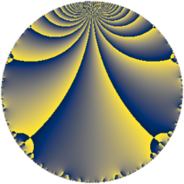# Properties

 Label 126.4.tLevel $126$ Weight $4$ Character orbit 126.t Rep. character $\chi_{126}(47,\cdot)$ Character field $\Q(\zeta_{6})$ Dimension $48$ Newform subspaces $1$ Sturm bound $96$ Trace bound $0$

# Related objects

## Defining parameters

 Level: $$N$$ $$=$$ $$126 = 2 \cdot 3^{2} \cdot 7$$ Weight: $$k$$ $$=$$ $$4$$ Character orbit: $$[\chi]$$ $$=$$ 126.t (of order $$6$$ and degree $$2$$) Character conductor: $$\operatorname{cond}(\chi)$$ $$=$$ $$63$$ Character field: $$\Q(\zeta_{6})$$ Newform subspaces: $$1$$ Sturm bound: $$96$$ Trace bound: $$0$$

## Dimensions

The following table gives the dimensions of various subspaces of $$M_{4}(126, [\chi])$$.

Total New Old
Modular forms 152 48 104
Cusp forms 136 48 88
Eisenstein series 16 0 16

## Trace form

 $$48q + 96q^{4} - 12q^{7} + 18q^{9} + O(q^{10})$$ $$48q + 96q^{4} - 12q^{7} + 18q^{9} - 72q^{13} - 132q^{14} + 132q^{15} - 384q^{16} - 144q^{17} - 72q^{18} + 270q^{21} + 48q^{24} + 1200q^{25} - 120q^{26} + 450q^{27} + 48q^{28} + 42q^{29} - 468q^{30} - 90q^{31} - 1332q^{33} + 780q^{35} - 240q^{36} - 168q^{37} + 240q^{39} + 618q^{41} - 252q^{42} - 42q^{43} - 96q^{44} + 1038q^{45} - 252q^{46} + 198q^{47} - 636q^{49} + 1464q^{50} + 222q^{51} + 36q^{53} + 180q^{54} - 1452q^{57} + 504q^{58} - 1500q^{59} - 480q^{60} + 2466q^{61} - 2952q^{62} - 2988q^{63} - 3072q^{64} - 84q^{65} - 384q^{66} - 588q^{67} - 1152q^{68} - 1848q^{69} + 216q^{70} + 192q^{72} + 2820q^{75} + 1548q^{77} + 2496q^{78} + 1230q^{79} - 4122q^{81} + 1512q^{84} + 720q^{85} + 258q^{87} + 4398q^{89} + 6084q^{90} - 90q^{91} - 1632q^{92} + 1740q^{93} + 3246q^{95} + 192q^{96} - 1584q^{97} + 1104q^{98} - 5076q^{99} + O(q^{100})$$

## Decomposition of $$S_{4}^{\mathrm{new}}(126, [\chi])$$ into newform subspaces

Label Dim. $$A$$ Field CM Traces $q$-expansion
$$a_2$$ $$a_3$$ $$a_5$$ $$a_7$$
126.4.t.a $$48$$ $$7.434$$ None $$0$$ $$0$$ $$0$$ $$-12$$

## Decomposition of $$S_{4}^{\mathrm{old}}(126, [\chi])$$ into lower level spaces

$$S_{4}^{\mathrm{old}}(126, [\chi]) \cong$$ $$S_{4}^{\mathrm{new}}(63, [\chi])$$$$^{\oplus 2}$$